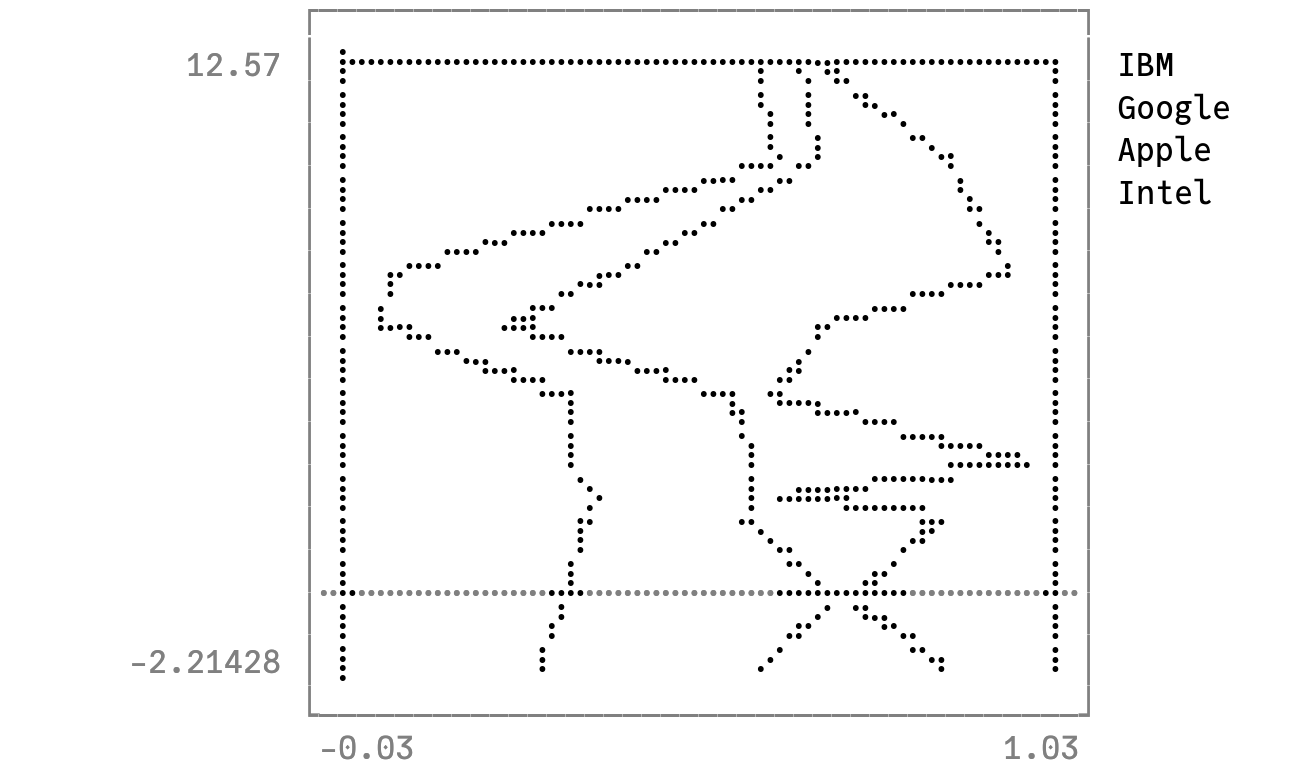# Portfolio Composition mapsusing Plots
unicodeplots()

see: https://stackoverflow.com/a/37732384

using Random
Random.seed!(111)
tickers = ["IBM", "Google", "Apple", "Intel"]
(N, D) = (10, length(tickers))
weights = rand(N, D)
weights ./= sum(weights, dims = 2)
returns = sort!((1:N) + D * randn(N))
portfoliocomposition(weights, returns, labels = permutedims(tickers))